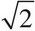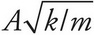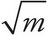# AP Physics 1 Question 248: Answer and Explanation

### Test Information

Question: 248

3. A student measures the maximum speed of a block undergoing simple harmonic oscillations of amplitude A on the end of an ideal spring. If the block is replaced by one with twice its mass but the amplitude of its oscillations remains the same, then the maximum speed of the block will

• A. decrease by a factor of 4
• B. decrease by a factor of 2
• C. decrease by a factor of• D. increase by a factor of 2

The maximum speed of the block is given by the equation vmax =. Therefore, vmax is inversely proportional to. If m is increased by a factor of 2, then vmax will decrease by a factor of.